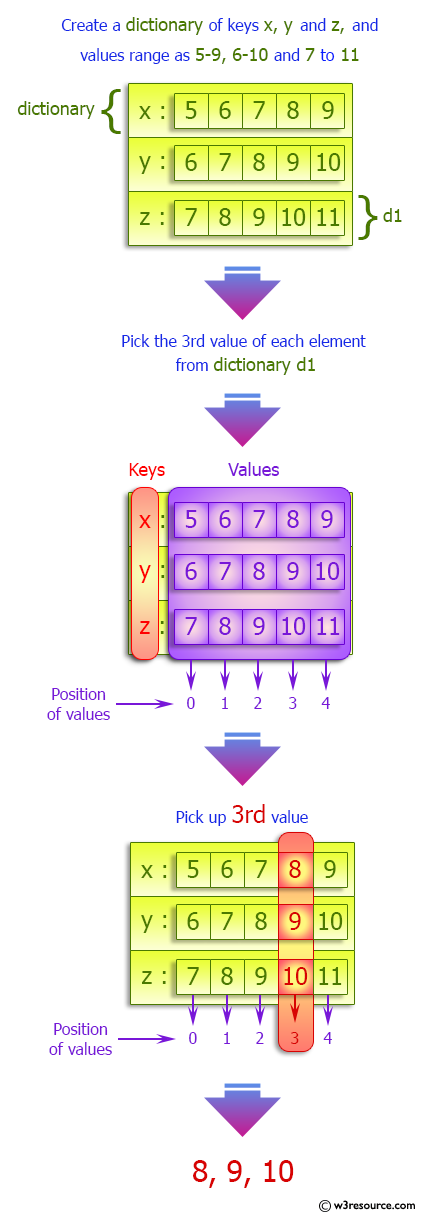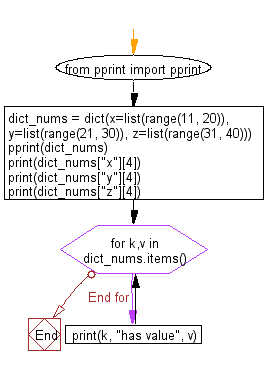﻿ Python: Create a dictionary of keys x, y, and z - w3resource# Python: Create a dictionary of keys x, y, and z

## Python dictionary: Exercise-40 with Solution

Write a Python program to create a dictionary of keys x, y, and z where each key has as value a list from 11-20, 21-30, and 31-40 respectively. Access the fifth value of each key from the dictionary.

Sample Solution:

Python Code:

``````from pprint import pprint
dict_nums = dict(x=list(range(11, 20)), y=list(range(21, 30)), z=list(range(31, 40)))
pprint(dict_nums)
print(dict_nums["x"])
print(dict_nums["y"])
print(dict_nums["z"])
for k,v in dict_nums.items():
print(k, "has value", v)
```
```

Sample Output:

```{'x': [11, 12, 13, 14, 15, 16, 17, 18, 19],
'y': [21, 22, 23, 24, 25, 26, 27, 28, 29],
'z': [31, 32, 33, 34, 35, 36, 37, 38, 39]}
15
25
35
x has value [11, 12, 13, 14, 15, 16, 17, 18, 19]
y has value [21, 22, 23, 24, 25, 26, 27, 28, 29]
z has value [31, 32, 33, 34, 35, 36, 37, 38, 39]
```

Pictorial Presentation:Flowchart:## Visualize Python code execution:

The following tool visualize what the computer is doing step-by-step as it executes the said program:

Python Code Editor:

Have another way to solve this solution? Contribute your code (and comments) through Disqus.

What is the difficulty level of this exercise?

Test your Programming skills with w3resource's quiz.

﻿

## Python: Tips of the Day

Invokes the provided function after ms milliseconds:

Example:

```from time import sleep

def tips_delay(fn, ms, *args):
sleep(ms / 1000)
return fn(*args)
print(tips_delay(
lambda x: print(x),
1000,
'w3r'
))
```

Output:

```w3r
None
```